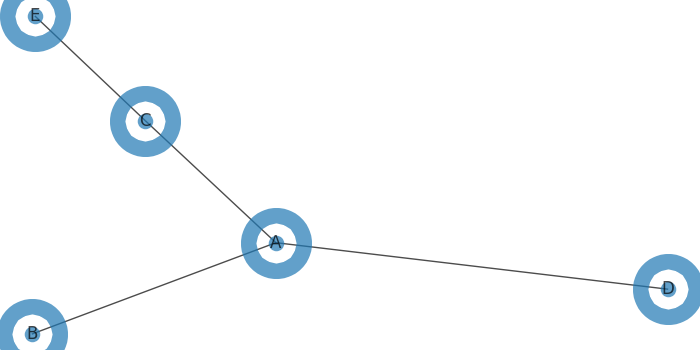# How to reshape a networkx graph in Python?

To reshape a networkx graph in Python, we can take the following steps −

• Create a data frame using Panda's data frame.

• Return a graph from Pandas DataFrame containing an edge list using from_pandas_edgelist() method.

• Draw the graph G with matplotlib. We can reshape the network by increasing and decreasing the list of keys "from" and "to".

• To display the figure, use show() method.

## Example

import pandas as pd
import networkx as nx
from matplotlib import pyplot as plt
plt.rcParams["figure.figsize"] = [7.00, 3.50]
plt.rcParams["figure.autolayout"] = True
df = pd.DataFrame({'from': ['A', 'B', 'C', 'A'], 'to': ['D', 'A', 'E', 'C']})
G = nx.from_pandas_edgelist(df, 'from', 'to')
nx.draw(G, with_labels=True, node_size=150, alpha=0.5, linewidths=40)
plt.show()

## Output